# IB DP Physics: SL复习笔记4.4.7 Double-Slit Equation

### Double-Slit Equation

#### Interference Patterns

• Interference patterns depend on:
• The coherent light incident on the slits
• The distance between the slits and the screen where the interference pattern is observed
• The separation between the slits
• In particular:
• Successive bright fringes are further apart from each other if the wavelength λ of the incident light is longer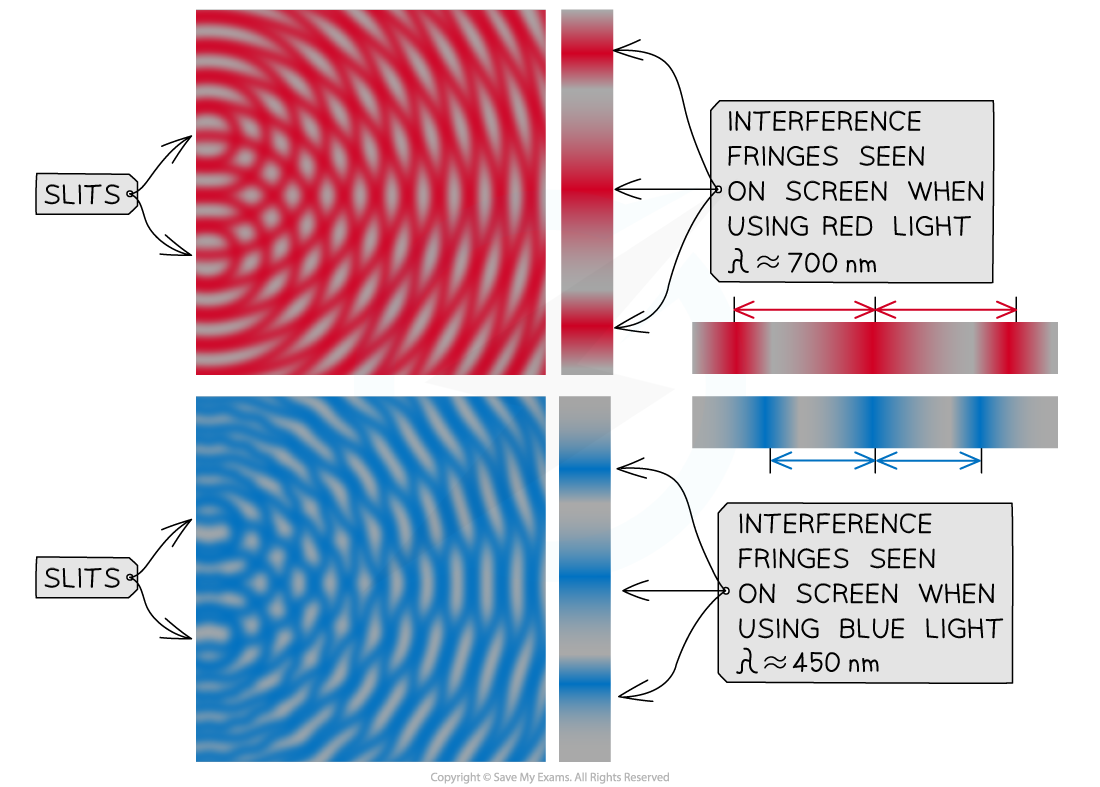Difference in the interference pattern of red and blue light. Red light has a longer wavelength than blue light, so the fringes are more spaced out

• Similarly, bright fringes are more spaced out if the screen is placed further away from the slits
• If the separation between the slits is increased, instead successive bright fringes are closer to each other

#### Explaining the Interference Pattern

• Two slits are separated by a length d, and a screen is placed a distance D away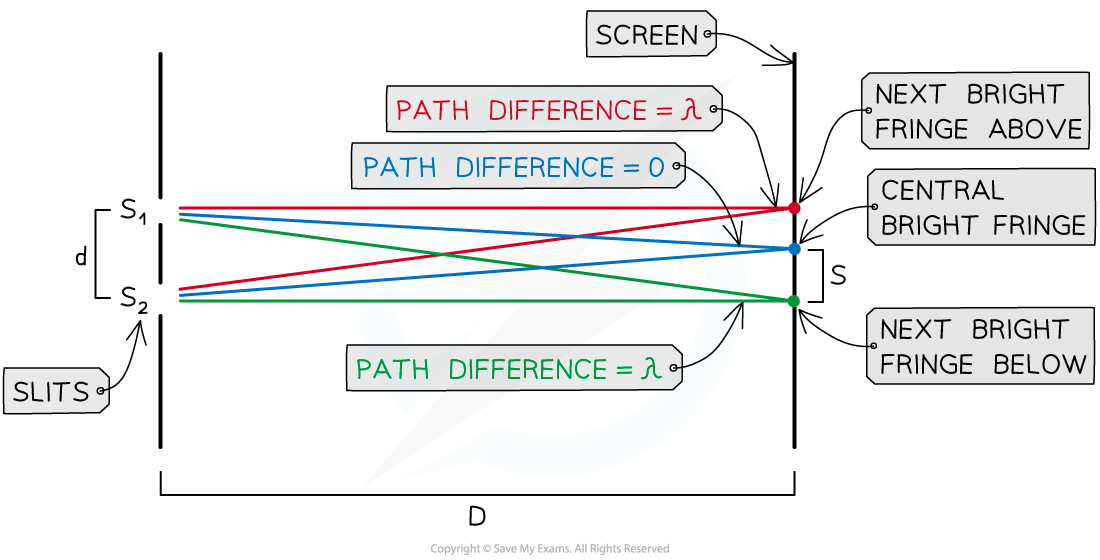Light rays of wavelength λ incident on two slits a distance s apart interferes and form bright fringes on a screen placed a distance D away from the slits

• When light rays of wavelength λ are incident on the two slits:
• A central bright fringe where the two waves have travelled the same distance to the screen and their path difference is zero
• Another bright fringe is formed either side of the central one where the path difference between the waves is  exactly one wavelength
• This is constructive interference
• Dark fringes (an absence of light) are formed where the path difference between the waves is some number of wavelengths plus half a wavelength
• This is destructive interference
• Further bright fringes will be located at each position on the screen where the path difference is exactly equal to n number of wavelengths nλ
• For example: 2λ, 3λ, 4λ...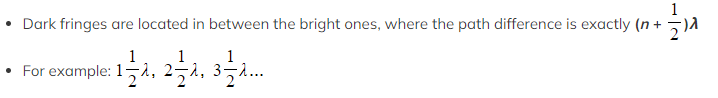Double-Slit Equation
• The separation s of successive fringes is given by: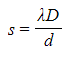• Where:
• s = separation between successive fringes on the screen (m)
• λ = wavelength of the waves incident on the slits (m)
• D = distance between the screen and the slits (m)
• d = separation between the slits (m)
• Note that s is the separation between two successive bright fringes or two successive dark ones
• The above equation shows that the separation between the fringes will increase if:
• The wavelength of the incident light increases
• The distance between the screen and the slits increases
• The separation between the slits decreases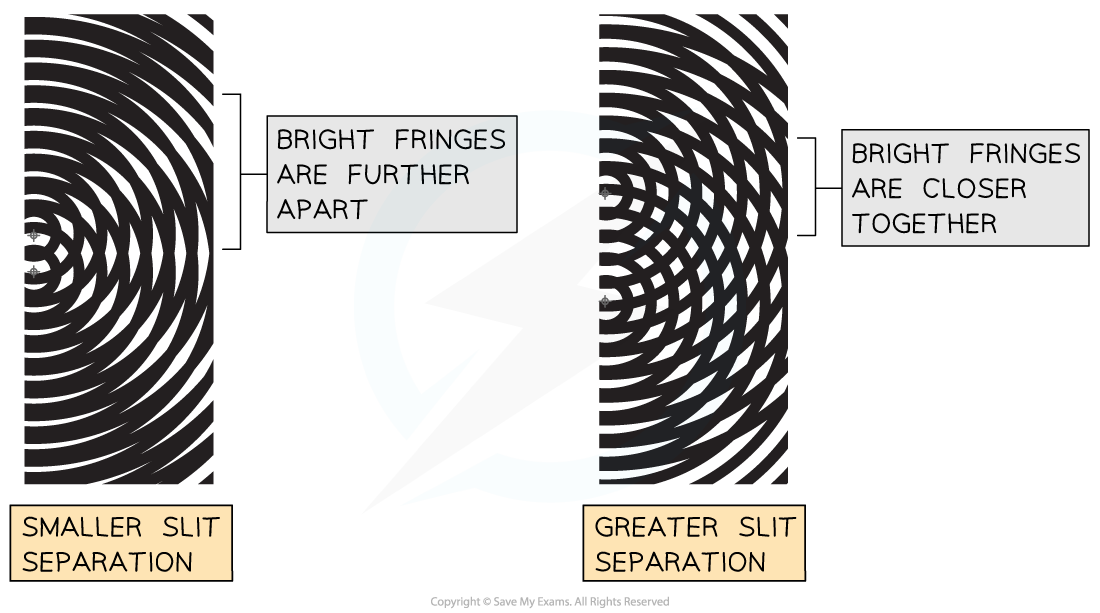Dependence of the interference pattern on the separation between the slits. The further apart the slits, the closer together the bright fringes

#### Worked Example

In a double-slit experiment, two slits are placed a distance of 0.40 mm apart and a screen is located 0.50 m away from the slits.

Coherent electromagnetic waves incident on the slits produce an interference pattern on the screen. The separation between dark fringes is 0.50 cm.

Determine the wavelength and state the type of electromagnetic waves used in the experiment.

Step 1: Write down the known quantities

• d = 0.40 mm = 4.0 x 10–4 m
• D = 0.50 m
• s = 0.50 cm = 5.0 x 10–3 m

Note that you must convert all lengths into metres (m)

Step 2: Write down the double-slit equation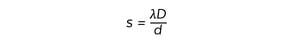Step 3: Rearrange the above equation to calculate the wavelength λ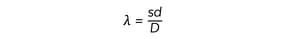Step 4: Substitute the numbers into the above equation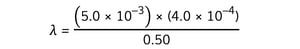λ = 4.0 × 10–6 m = 4.0 μm

This corresponds to the infrared area of the electromagnetic spectrum

#### Exam Tip

Remember that the separation between dark fringes is exactly the same as the separation between bright fringes. Whether a question gives you or asks about the separation between dark fringes instead of bright ones it makes no difference.

Some tricky questions might give you the separation between a bright and a dark fringe. This is equal to half the value of s!